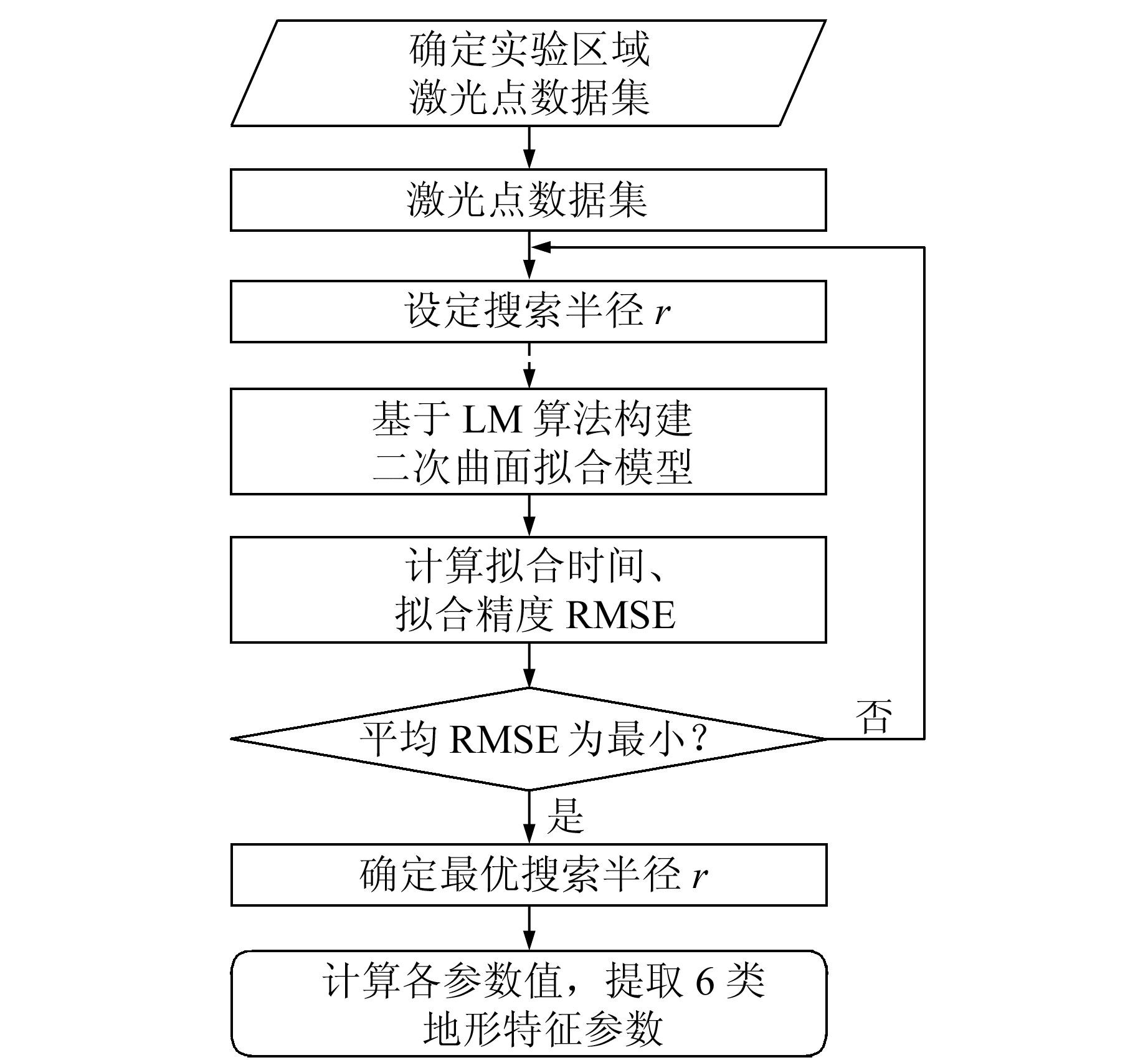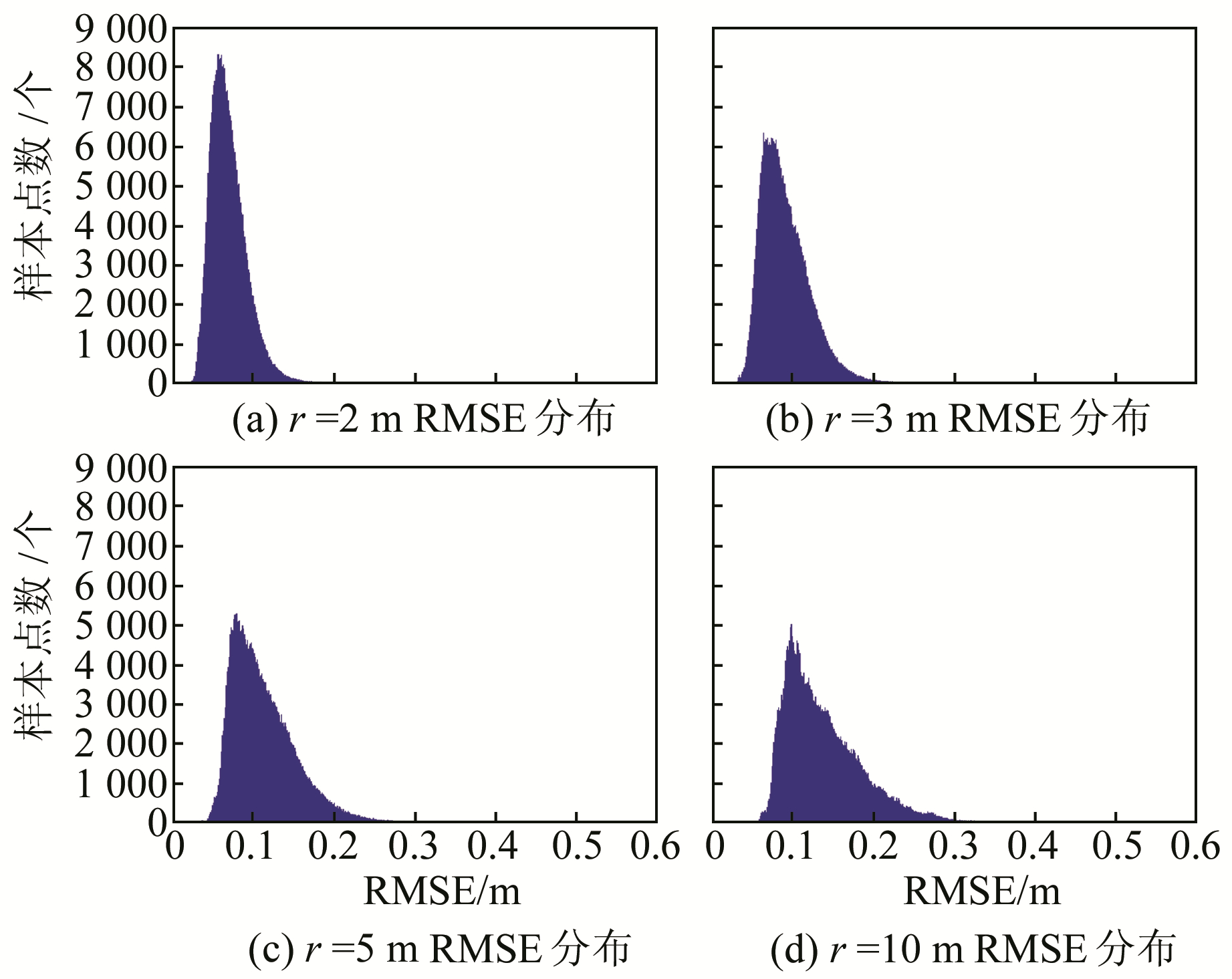﻿ 机载LiDAR地形特征信息快速提取文章快速检索 高级检索
 大地测量与地球动力学2018, Vol. 38Issue (11): 1186-1190, 1206  DOI: 10.14075/j.jgg.2018.11.017### 引用本文LIU Zhimin, YANG Anxiu, SU Dianpeng, et al. Terrain Features Rapid Extraction of Airborne LiDAR[J]. Journal of Geodesy and Geodynamics, 2018, 38(11): 1186-1190, 1206.### Foundation support

National Natural Science Foundation of China, No. 41374009; Open Fund of Key Laboratory of Surveying and Mapping Technology on Island and Reef, NASMG, No. 2015B01.

### About the first author

LIU Zhimin, PhD, associate professor, majors in the theories of GNSS and marine survey, E-mail: liuzhimin010@163.com.

### 文章历史

1. 山东科技大学测绘科学与工程学院, 青岛市前湾港路579号, 266590;
2. 海岛(礁)测绘技术国家测绘地理信息局重点实验室, 青岛市前湾港路579号, 266590

1 地形特征信息提取表 1 地形特征参数描述 Tab. 1 Description of the terrain feature variables图 1 部分地形特征示意图 Fig. 1 Schematic illustration of the part of terrain feature variables图 2 地形特征参数提取流程 Fig. 2 Flowchart of the terrain features extraction

6类地形特征参数中，局部点密度和高程标准差不再详细叙述，坡度、曲率(高斯曲率、平均曲率)和粗糙度的提取过程如下。

1.1 二次曲面拟合

 $z = f\left( {x,y} \right) = a{x^2} + b{y^2} + cxy + dx + ey + f$ (1)

 $\begin{array}{l} {Q^2} = \min \sum\limits_{j = 1}^k {\left[ {{z_j} - \left( {ax_j^2 + by_j^2 + } \right.} \right.} \\ \;\;\;\;{\left. {\left. {c{x_j}{y_j} + d{x_j} + e{y_j} + f} \right)} \right]^2} \end{array}$ (2)

 $\left\{ \begin{array}{l} \frac{{\partial {Q^2}}}{{\partial a}} = 0,\frac{{\partial {Q^2}}}{{\partial b}} = 0\\ \frac{{\partial {Q^2}}}{{\partial c}} = 0,\frac{{\partial {Q^2}}}{{\partial d}} = 0\\ \frac{{\partial {Q^2}}}{{\partial e}} = 0,\frac{{\partial {Q^2}}}{{\partial f}} = 0 \end{array} \right.$ (3)

 $\mathit{\boldsymbol{AX}} = \mathit{\boldsymbol{B}}$ (4)

 $\mathit{\boldsymbol{X}} = {\mathit{\boldsymbol{A}}^{ - 1}}\mathit{\boldsymbol{B}}$ (5)

1.2 坡度计算

 $\varphi \mathit{\boldsymbol{ = }}{\rm{arctan}}\sqrt {{{\tan }^2}{\varphi _x} + {{\tan }^2}{\varphi _y}}$ (6)

 $\left[ {\begin{array}{*{20}{c}} {\tan {\varphi _x}}\\ {\tan {\varphi _y}} \end{array}} \right] = \left[ {\begin{array}{*{20}{c}} p\\ q \end{array}} \right],\left\{ \begin{array}{l} p = \frac{{\partial z}}{{\partial x}} = 2ax + cy + d\\ q = \frac{{\partial z}}{{\partial y}} = 2by + cx + e \end{array} \right.$
1.3 曲率计算

 $\begin{array}{*{20}{c}} {\left( {rt - {s^2}} \right){R^2} + h\left[ {2pqs - \left( {1 + {p^2}} \right)t - } \right.}\\ {\left. {\left( {1 + {q^2}} \right)r} \right]R + {h^4} = 0} \end{array}$ (7)

 $\left. \begin{array}{l} {C_{\max }} = \frac{1}{{{R_{\max }}}}\\ {C_{\min }} = \frac{1}{{{R_{\min }}}} \end{array} \right\}$ (8)

 ${C_{\rm{G}}} = {C_{\max }}{C_{\min }} = \frac{{rt - {s^2}}}{{{{\left( {1 + {p^2} + {q^2}} \right)}^2}}}$ (9)

 $\begin{array}{*{20}{c}} {{C_{\rm{m}}} = \frac{1}{2}\left( {{C_{\max }} + {C_{\min }}} \right) = }\\ { - \frac{{\left( {1 + {q^2}} \right)r - 2pqs + \left( {1 + {p^2}} \right)t}}{{2{{\left( {1 + {p^2} + {q^2}} \right)}^{\frac{3}{2}}}}}} \end{array}$ (10)
1.4 地形粗糙度计算

 $\begin{array}{*{20}{c}} {{K_{\text{r}}} = \frac{{{S_S}}}{{{S_E}}} = \frac{{\iint\limits_E {\sqrt {\left( {1 + {p^2} + {q^2}} \right)} {\text{d}}x{\text{d}}y}}}{{{S_E}}} = } \\ {\frac{{\int_{{x_{\min }}}^{{x_{\max }}} {\int_{{y_{\min }}}^{{y_{\max }}} {\sqrt {\left( {1 + {p^2} + {q^2}} \right)} {\text{d}}x{\text{d}}y} } }}{{{S_E}}}} \end{array}$ (11)
 ${S_E} = \frac{1}{2}\left( {\left| {\begin{array}{*{20}{c}} {{x_1}}&{{y_1}}\\ {{x_2}}&{{y_2}} \end{array}} \right| + \left| {\begin{array}{*{20}{c}} {{x_2}}&{{y_2}}\\ {{x_3}}&{{y_3}} \end{array}} \right| + \cdots + \left| {\begin{array}{*{20}{c}} {{x_n}}&{{y_n}}\\ {{x_1}}&{{y_1}} \end{array}} \right|} \right)$ (12)

2 实验与分析 2.1 研究数据图 3 研究数据 Fig. 3 Study data
2.2 拟合精度分析表 2 不同搜索半径的曲面拟合结果 Tab. 2 The surface fitting results of different search radius图 4 不同搜索半径r的RMSE分布直方图 Fig. 4 RMSE distribution histograms of different r

2.3 地形特征提取结果分析图 5 地形特征向量提取结果 Fig. 5 Results of terrain features vector extraction

3 结语

  刘经南, 张小红. 激光扫描测高技术的发展与现状[J]. 武汉大学学报:信息科学版, 2003, 28(2): 132-137 (Liu Jingnan, Zhang Xiaohong. Progress of Airborne Laser Scanning Altimetry[J]. Geomatics and Information Science of Wuhan University, 2003, 28(2): 132-137) (0)  Zhang J, Zhang L, Zeng F, et al. Development Status of Airborne 3D Imaging LiDAR System[J]. Chinese Journal of Optics and Applied Optics, 2011, 4(3): 213-232 (0)  Lohmann P, Koch A. Quality Assessment of Laser-scanner-data[C].The International Society of Photogrammetry and Remote Sensing (ISPRS), Hannover, Germany, 1999 (0)  Susaki J. Adaptive Slope Filtering of Airborne LiDAR Data in Urban Areas for Digital Terrain Model (DTM) Generation[J]. Remote Sensing, 2012(4): 1 804-1 819 (0)  Park B, Kim J, Lee J. Terrain Feature Extraction and Classification for Mobile Robots Utilizing Contact Sensors on Rough Terrain[J]. Procedia Engineering, 2012, 41: 846-853 DOI:10.1016/j.proeng.2012.07.253 (0)  Matthies L, Huertas A, Yang C, et al.Landing Hazard Detection with Stereo Vision and Shadow Analysis[R]. AIAA-2007-2835, 2007 http://www.mendeley.com/research/stereo-vision-shadow-analysis-landing-hazard-detection/ (0)  王强锋, 李建军, 张公平. 从随机非概率数据中提取粗糙度及其可靠度[J]. 计算机工程与应用, 2012, 48(29): 168-171 (Wang Qiangfeng, Li Jianjun, Zhang Gongping. Research of Extraction of Target Roughness and Its Reliability from Randomly Non-probabilistic Data[J]. Computer Engineering and Applications, 2012, 48(29): 168-171 DOI:10.3778/j.issn.1002-8331.2012.29.034) (0)  Hollaus M, Aubrecht C, Höfle B, et al. Roughness Mapping on Various Vertical Scales Based on Full-Waveform Airborne Laser Scanning Data[J]. Remote Sensing, 2011, 3(3): 503-523 DOI:10.3390/rs3030503 (0)  Klingseisen B, Metternicht G, Paulus G. GeomorphometricLandscape Analysis Using a Semi-Automated GIS-Approach[J]. Environmental Modeling & Software, 2008, 23(1): 109-121 (0)  张蓉.散乱点云的数据分割与特征提取技术研究[D].南昌: 南昌大学, 2016 (Zhang Rong. Research on Data Segmentation and Feature Extraction Techniques of Scattered[D]. Nanchang: Nanchang University, 2016) http://cdmd.cnki.com.cn/Article/CDMD-10403-1016256520.htm (0)  Yi D, Zwally H J, Sun X. ICESat Measurement of Greenland Ice Sheet Surface Slope and Roughness[J]. Annals of Glaciology, 2005, 42(1): 86-89 (0)  杨荣华, 花向红, 游扬声. 基于散乱点的局部n次曲面拟合及其曲率计算[J]. 大地测量与地球动力学, 2013, 33(3): 141-144 (Yang Ronghua, Hua Xianghong, You Yangsheng. Local N-th Order Surface Fitting and Curvature Estimation Based on Scattered-Point Cloud Data[J]. Journal of Geodesy and Geodynamics, 2013, 33(3): 141-144) (0)  Shary P A. Land Surface in Gravity Points Classification by a Complete System of Curvatures[J]. Mathematical Geology, 1995, 27(3): 373-390 DOI:10.1007/BF02084608 (0)  Shary P A, Sharaya L S, Mitusov A V. Fundamental Quantitative Methods of Land Surface Analysis[J]. Geoderma, 2002, 107(1-2): 1-32 DOI:10.1016/S0016-7061(01)00136-7 (0)  Mukherjee S, Mukherjee S, Garg R D, et al. Evaluation of Topographic Index in Relation to Terrain Roughness and DEM Grid Spacing[J]. Journal of Earth System Science, 2013, 122(3): 869-886 DOI:10.1007/s12040-013-0292-0 (0)
Terrain Features Rapid Extraction of Airborne LiDAR
LIU Zhimin1,2YANG Anxiu1     SU Dianpeng1     LIU Pan1
1. College of Geomatics, Shandong University of Science and Technology, 579 Qianwangang Road, Qingdao 266590, China;
2. Key Laboratory of Surveying and Mapping Technology on Island and Reef, NASMG, 579 Qianwangang Road, Qingdao 266590, China
Abstract: Terrain features play an important role in cartography, earthquake geology, terrain classification and DEM production. In order to obtain terrain features more effectively, a rapid terrain features extraction algorithm of airborne LiDAR is proposed in this paper. Firstly, constructing the quadric surface fitting model, the geometric rules of airborne LiDAR data and fitting model are established. Then, the LM(levenberg-marquardt) algorithm is adopted to optimize the parameters of the topography and obtain the optimal results. In addition, the fitting time and fitting precision are calculated. Finally, the terrain features are extracted rapidly based on the terrain fitting model. To verify the algorithm's efficiency and accuracy, the airborne LiDAR experiment indicates that when the optimal search radius is 2 m, the fitting time is only 0.02 s and RMSE is only 5.09 cm.
Key words: airborne LiDAR; levenberg-marquardt fitting; curvature; roughness; slope